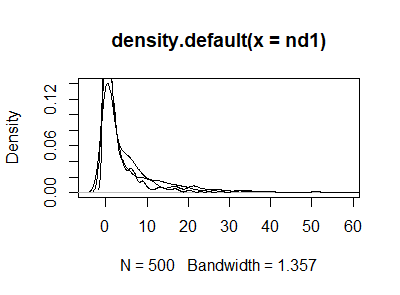# #StackBounty: #modeling #simulation #negative-binomial How to create average model of multiple negative binomial models?

### Bounty: 50

I have 40 datasets, i manage to fit them to negative binomial model with different model parameters (size and mu). I am trying (if this is possible) to get resultant model that will replace "reasonable well" all this models with "default" one. I am planing to make simulation and want to make model for that category of users. Can I use mean values from all model for size and mu for this resultant model. So question is how can I create average model?

example

``````nd1 <- rnbinom(500, size=0.406, mu = 5.398)
nd2 <- rnbinom(500, size=0.389, mu = 2.544)
nd3 <- rnbinom(500, size=0.351, mu = 4.124)
``````

density curvesNow I want "average" for all this datasets, to produce resultant model. Hope that this explain what I am trying to get.

I used average for mu and size, size = (0.406 + 0.389 + 0.351)/3 = 0,382; mu = 5.389 + 2.544 + 4.124)/3 = 4,019, I know that this is real simple approach, is there some other method. My data contain one variable and no predictors.

``````ndres <- rnbinom(500, size=0.389, mu = 4.019)
plot(density(nd1))
lines(density(nd2))
lines(density(nd3))
lines(density(ndres),col="red")
``````

Get this bounty!!!

This site uses Akismet to reduce spam. Learn how your comment data is processed.